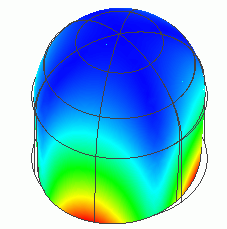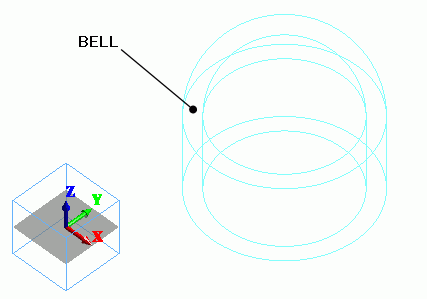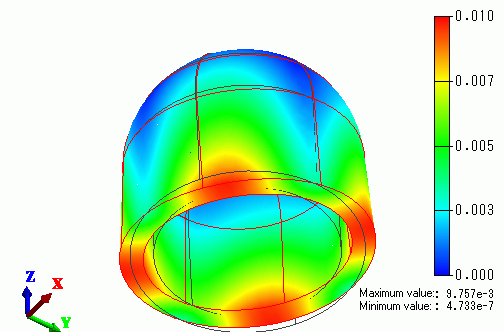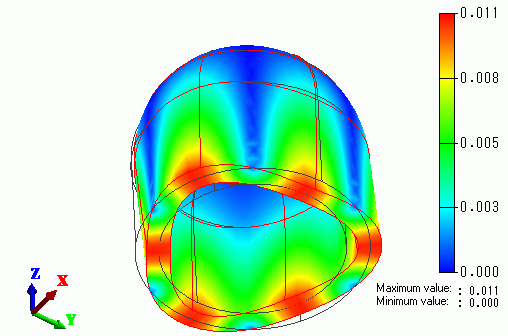﻿ Resonance of Church BellExamples | Product | Murata Software Co., Ltd.Example11Resonance of Church BellGeneral

• The resonance of a church bell is analyzed.

• The deformation, the displacement and the mechanical stress are solved.

• Unless specified in the list below, the default conditions will be applied.

Analysis Space

 Item Settings Analysis Space 3D Model unit m

Analysis Conditions

The analysis type is the resonant analysis.

 Item Settings Solver Mechanical Stress Analysis [Galileo] Analysis Type Resonant analysis Options N/A

The resonant analysis tab is set up as follows.

 Tabs Setting Item Settings Resonant analysis Number of modes 6 Approximated frequency 0[Hz]

Model

Two spherical solid bodies and two cylindrical solid bodies are created, first. The smaller sphere and cylinder, and the larger sphere and cylinder are united by boolean respectively.

The smaller object is subtracted from the larger one. As a result, a church bell is created.Body Attributes and Materials

 Body Number/Type Body Attribute Name Material Name 10/Solid BELL 008_Cu *

* Available from the Material DB

N/A

Results

The following will be output on the output window or the log file.

 <> Eigenvalue (resonant frequency):[Hz] Mode[ 0] = 8.61317565e+001 Mode[ 1] = 8.61493565e+001 Mode[ 2] = 2.25520570e+002 Mode[ 3] = 2.26128750e+002 Mode[ 4] = 3.22020390e+002 Mode[ 5] = 3.22035453e+002

The resonant frequencies can be checked on Table.

The fundamental resonant frequency is 86.1[Hz].

There are two degenerated fundamental resonant modes, which are Mode and Mode.

The displacement diagram of Mode is shown below. The contour diagram shows the displacement.The displacement diagram of Mode is shown below. The contour diagram shows the displacement.The number of nodes are 4 in the fundamental mode, whereas it is 6 in the higher-order mode.

﻿